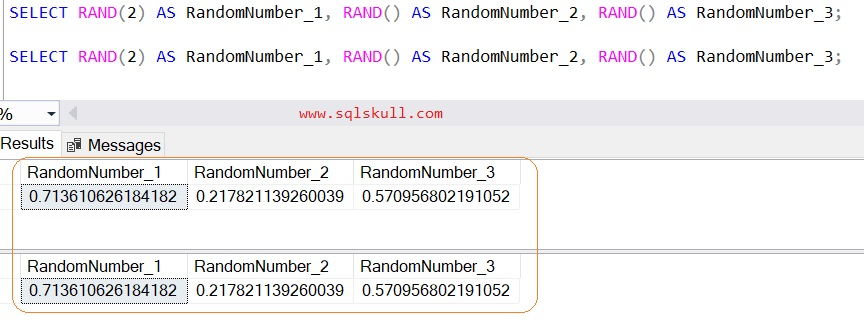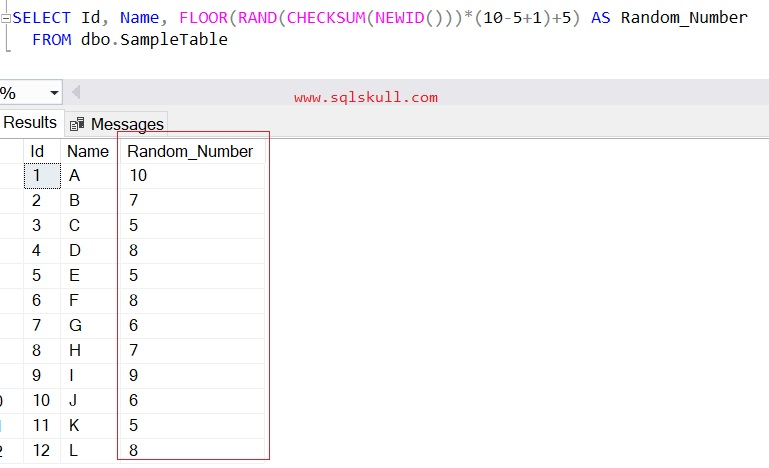Home » SQL Random Number

# SQL Random NumberSQL RAND function is a mathematical function which returns a pseudo-random float value from 0 through 1, exclusive or a random value within any range.

It can also take an optional seed parameter which is an integer expression (tinyint, smallint or int) that gives the seed.

SYNTAX

`RAND ( [ seed ] )`

seed
Is an integer expression (tinyint, smallint, or int) that gives the seed value. For a specified seed value, it always return the same result,  If seed is not specified, then RAND function assigns a seed value at random. It is an optional.

Lets look at an example of using RAND function in SQL Server.

#### Generating random number using RAND function

Following statement uses RAND function which returns a random number between range > 0 and < 1.

Executing the same statement on multiple times, you will see each time RAND function returns random number between the range > 0 and < 1.  as shown below.

```SELECT RAND() AS RandomNumber_1;
SELECT RAND() AS RandomNumber_2;
SELECT RAND() AS RandomNumber_3;

```#### Specifying a seed value to RAND Function

When a seed value is specified seed value to RAND function, it always return the same result.

In following statement a seed value 2 is specified to RAND function, you can see when executing the same statement for multiple times with 2 as the seed of the RAND function will always returns same result.

```SELECT RAND(2) AS RandomNumber_1;
SELECT RAND(2) AS RandomNumber_2;
SELECT RAND(2) AS RandomNumber_3;```Also note that, If seed value is specified then all subsequent calls of RAND function returns results based on the seeded RAND function call as shown below.

```SELECT RAND(2) AS RandomNumber_1,
RAND() AS RandomNumber_2,
RAND() AS RandomNumber_3;

SELECT RAND(2) AS RandomNumber_1,
RAND() AS RandomNumber_2,
RAND() AS RandomNumber_3;```You can see after specifying the seed values to RAND function, all subsequent calls of RAND function returns results based on the seeded RAND function call, also returns same result on multiple execution.

#### Generating random integer number between range

You can also use RAND function for generating a random integer number between any specified range, inclusive, or you can say that generating a random number between min and max values, inclusive.

Also note that, for any specified range, a random number could be repeat.

Following is the syntax that you can use to generate a random integer values between any specified range(min and max value).

`SELECT FLOOR(RAND()*(max_Value- min_value +1))+ min_value;`

Lets generate random integer number between range 5 to 10, inclusive.

For given range 5 to 10, value 5 is considered as min_value while 10 is considered as max_value.

```SELECT FLOOR(RAND()*(10- 5))+ 5 AS Random_Number1
SELECT FLOOR(RAND()*(10- 5))+ 5 AS Random_Number2
SELECT FLOOR(RAND()*(10- 5))+ 5 AS Random_Number3
SELECT FLOOR(RAND()*(10- 5))+ 5 AS Random_Number4```You can see, it returns a random number for specified range 5 to 10.

#### Generating Random decimal number between range

You can also use RAND function for generating a random decimal number between any specified range, exclusive, or you can say that generating a random number between min and max values, exclusive.

Following is the syntax that you can use to generate a random decimal values between any specified range(min and max value).

`SELECT RAND()*(Max_Value- Min_Value)+ Min_Value;`

Lets generate random integer number between range 5 to 10, exclusive.

For given range 5 to 10, value 5 is considered as min_value while 10 is considered as max_value.

```SELECT RAND()*(10- 5)+ 5 AS Random_Number1
SELECT RAND()*(10- 5)+ 5 AS Random_Number2
SELECT RAND()*(10- 5)+ 5 AS Random_Number3
SELECT RAND()*(10- 5)+ 5 AS Random_Number4```You can see, it returns random decimal number for specified range 5 to 10, exclusive.

#### RAND function while fetching a data from table

When you specified a RAND function in any single SELECT statement on a table then the value returned for each row will be the same.

To demonstrate this, Lets create a table and insert some dummy records into that table, as shown below.

```CREATE TABLE dbo.ampleTable
(ID INT Identity(1,1),
Name VARCHAR(10)
)

INSERT INTO dbo.SampleTable
(Name) VALUES
('A'),('B'),('C'),('D'),
('E'),('F'),('G'),('H'),
('I'),('J'),('K'),('L')```

Assume that, you want to generate a random integer number for each records in table while fetching the data from a table.

Lets say you want random integer number between range 5 to 10, inclusive.

```SELECT Id, Name, FLOOR(RAND()*(10- 5))+ 5 AS Random_Number
FROM dbo.SampleTable```You can see, it returns same random number for each records in table.

If you do want same random number for each records then you can modify above statement as shown below.

```SELECT Id,
Name,
FLOOR(RAND(CHECKSUM(NEWID()))*(10-5+1)+5) AS Random_Number
FROM dbo.SampleTable```You can see, this time it returns a random number for each rows, also you can see there are some duplicate random number as rand function can generate duplicate number for given range.